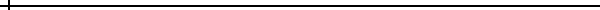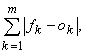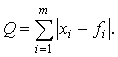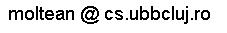# Traceless Genetic ProgrammingStructure

1.    Prerequisite

The aim of symbolic regression is to find a mathematical expression that satisfies best a set of fitness cases. Each fitness case is given as a (n+1) dimensional array of real values:

x1, x2, x3, …, xn, f1

where x1, x2, x3, …, xn are the values of the problem's attributes and f is the target value.

Usually more fitness cases are given (let us denote by m this number) and the task is to find the expression that satisfies best all these fitness cases. This is usually done by minimizing the quantity:where fk is the target value for the kth fitness case and ok is the actual (obtained) value for the kth fitness case.

2.    Individual representation

Each TGP individual represents a mathematical expression evolved so far, but the TGP individual does not explicitly store this expression. Each TGP individual stores only the obtained value so far for each fitness case. Thus a TGP individual is:

(o1, o2, o3, …, om)T,

where ok is the current value for the kth fitness case. Each position in this array (a value ok) is a gene. As we said it before behind these values is a mathematical expression whose evaluation has generated these values. However, we do not store this expression. We store only the values ok.

3.    Initial population

The initial population contains individuals whose values have been generated by simple expressions (made of a single terminal). For instance if an individual in the initial population represent the expression

E = x1,

then this individual is represented as:

C = (x1, x2, …, xm)T

where xk is the value of the first attribute for the kth fitness case.

The quality of this individual is4.    Genetic Operators

TGP uses two genetic operators: crossover and insertion.

4.1.    Crossover

For obtaining new individuals the crossover operator comes into role. For crossover several individuals (the parents) and a function symbol are selected. The offspring is obtained by computing by applying the selected operator for each of the genes of the parents.

The number of parents selected for crossover depends on the number of arguments required by the selected function symbol. If the function symbol is a binary operator then two parents have to be selected for crossover. If the function symbol is a unary operator, then a single parent needs to be selected.

Example

Let us suppose that the + operator is selected. In this case two parents

C1 = (p1, p2, …, pm)T  and

C2 = (q1, q2, …, qm)T

are selected and the offspring O is obtained as follows:

O = (p1+q1, p2+q2,…, pm+qm)T.

Example 2

Let us suppose that the sin operator is selected. In this case one parent

C1 = (p1, p2, …, pm)T

is selected and the offspring O is obtained as follows:

O = (sin(p1), sin(p2),…, sin(pm))T.

4.2.    Insertion

This operator inserts a simple expression (made of a single terminal) in the population. This operator is useful when the population contains individuals representing highly complex expressions that are not anymore able to improve the search. By inserting simple expressions we give a chance to the evolutionary process to choose another direction for evolution.

5.    TGP Algorithm

Due to the special representation and due to the newly proposed genetic operators, TGP uses a specific algorithm which is given below:

The TGP algorithm starts by creating a random population of individuals. The following steps are repeated until a given number of generations is reached: Two parents are selected using a standard selection procedure. The parents are recombined in order to obtain two offspring. The offspring are considered for mutation. The best offspring O replaces the worst individual W in the current population if O is better than W.

The variation operators ensure that the chromosome length is a constant of the search process. The algorithm returns as its answer the best expression evolved along a fixed number of generations.

The standard TGP algorithm is outlined below:

#### Standard TGP Algorithm

S1. Randomly create the initial population P(0)

S2. for t = 1 to NumberOfGenerations do

S3.   P’(t) = f

S4.   Copy the best individual from P(t) to P’(t)

S5.   while P’(t) is not filled do

S6.     with a fixed insertion probability pinsert do

Randomly select an input vector and copy it to P’(t)

S7.     with a crossover probability 1 – pinsert do

S8.       Select an operator.

S9.       Select a number of parents equal to the number of arguments of the selected operator.

S10.     Crossover the selected parents. An offspring offspr is obtained

S11.      Add the offspring o to P’(t)

S12.  endwhile

S13.  P(t+1) = P’(t);

S14. endforHome | People | TGP description | Papers | Source Code | Links   contact:Last updated: miercuri mai 03, 2006.